## RS Aggarwal Class 6 Solutions Chapter 21 Concept of Perimeter and Area Ex 21A

These Solutions are part of RS Aggarwal Solutions Class 6. Here we have given RS Aggarwal Solutions Class 6 Chapter 21 Concept of Perimeter and Area Ex 21A

Other Exercises

Question 1.
Solution:
(i) Length (l) = 16.8 cm
Perimeter = 2 (l + b)
= 2 (16.8 + 6.2) cm
= 2 x 23
= 46 cm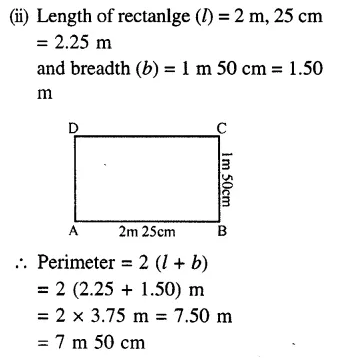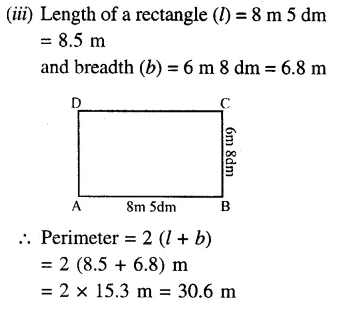= 30m 6 dm

Question 2.
Solution:
Length of rectangular field (l) = 62 m
and breadth (b) = 33 m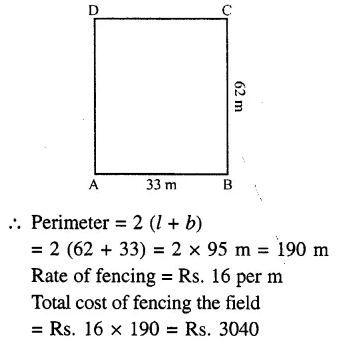Question 3.
Solution:
Perimeter of field = 128 m
Length + Breadth = $$\\ \frac { 128 }{ 2 }$$ = 64 m
Ratio in length and breadth = 5:3
Let length (l) = 5x
5x + 3x = 64
=> 5x = 64
=> x = $$\\ \frac { 64 }{ 8 }$$ = 8
Length of the field = 5x = 5 x 8 = 40m
and breadth = 3x = 3 x 8 = 24m

Question 4.
Solution:
Cost of fencing a rectangular field
= Rs. 18 per m
Total cost = Rs. 1980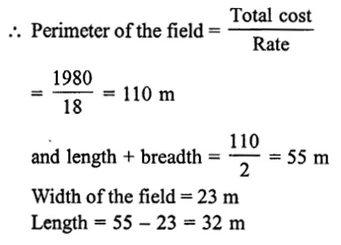Question 5.
Solution:
Total cost of fencing a rectangular field
= Rs 3300
Rate of fencing = Rs 25 per mQuestion 6.
Solution:
(i) Side of square = 3.8 cm
Perimeter = 4 x side
= 4 x 3.8 cm
= 15.2 cm
(ii) Side of a square = 4.6 m
Perimeter = 4 x side
= 4 x 4.6 m
= 18.4 m
(iii) Side of a square = 2 m 5 dm
= 2.5 m
Perimeter = 4 x side
= 4 x 2.5 m
= 10 m

Question 7.
Solution:
Total cost of fencing a square field = Rs. 4480
Rate of fencing = Rs. 35 per m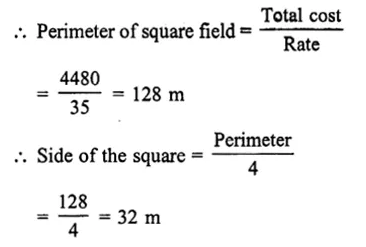Question 8.
Solution:
Side of a square field (a) = 21 m
Perimeter = 4a = 4 x 21 = 84m
Perimeter of rectangular field = 84 m
Ratio in length and breadth = 4 : 3
Let length (l) = 4x
Perimeter = 2 (l + b)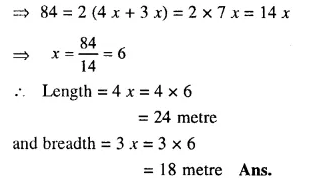Question 9.
Solution:
(i) Sides of a triangle are 7.8 cm, 6.5 cm and 5.9 cm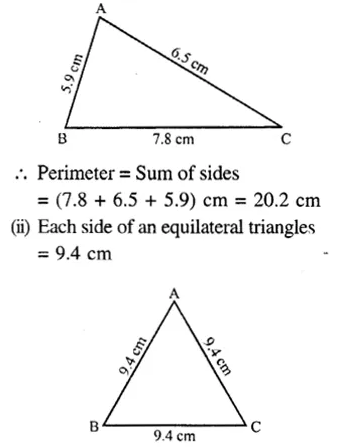Question 10.
Solution:
(i) Each side of a regular pentagon
= 8 cm
Perimeter = 5 x Side
= 5 x 8
= 40 cm
(ii) Each side of an octagon = 4.5 cm
Perimeter = 8 x Side
= 8 x 4.5
= 36 cm
(iii) Each side of a regular decagon = 3.6 cm
Perimeter = 10 x Side
= 10 x 3.6
= 36 cm

Question 11.
Solution:
We know that perimeter of a closed figure or a polygon = Sum of its sides
(i) In the figure, sides of a quadrilateral are 45 cm, 35 cm, 27 cm, 35 cm
Its perimeter = Sum of its sides
= (45 + 35 + 27 + 35) cm
= 142 cm
(ii) Sides of a quadrilateral (rhombus) are 18 cm, 18 cm, 18 cm, 18 cm
i.e., each side = 18 cm Perimeter
= 4 x Side
= 4 x 18
= 72 cm
(iii) Sides of the polygon given are 16 cm, 4 cm, 12 cm, 12 cm, 4 cm, 16 cm and 8 cm
Its perimeter = Sum of its sides
= (16 + 4 + 12 + 12 + 4 + 16 + 8) cm
= 72 cm

Hope given RS Aggarwal Solutions Class 6 Chapter 21 Concept of Perimeter and Area Ex 21A are helpful to complete your math homework.

If you have any doubts, please comment below. Learn Insta try to provide online math tutoring for you.